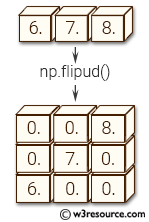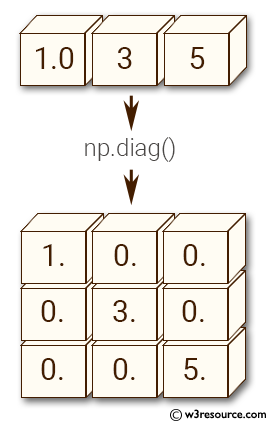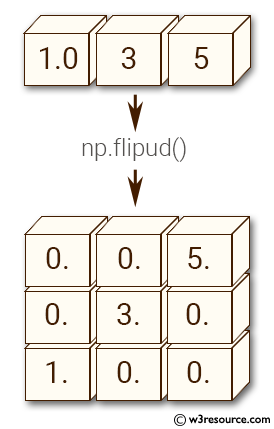# NumPy Array manipulation: flipud() function

## numpy.flipud() function

The flipud() function is used to flip an given array in the up/down direction.
Flip the entries in each column in the up/down direction. Rows are preserved, but appear in a different order than before.

Syntax:

numpy.flipud(m)Version: 1.15.0

Parameter:

Name Description Required /
Optional
m Input array. Required

Return value:

out : array_like - A view of m with the rows reversed. Since a view is returned, this operation is \mathcal O(1).

Example-1: numpy.flipud() function

>>> import numpy as np
>>> X = np.diag([1.0, 3, 5])
>>> X
array([[ 1.,  0.,  0.],
[ 0.,  3.,  0.],
[ 0.,  0.,  5.]])


Pictorial Presentation:Example-2: numpy.flipud() function

>>> import numpy as np
>>> X = np.diag([1.0, 3, 5])
>>> np.flipud(X)
array([[ 0.,  0.,  5.],
[ 0.,  3.,  0.],
[ 1.,  0.,  0.]])


Pictorial Presentation:Example-3: numpy.flipud()

>>> import numpy as np
>>> X = np.random.randn(3,5,7)
>>> np.all(np.flipud(X) == X[::-1,...])
True


Python - NumPy Code Editor:

Previous: fliplr()
Next: roll()

﻿